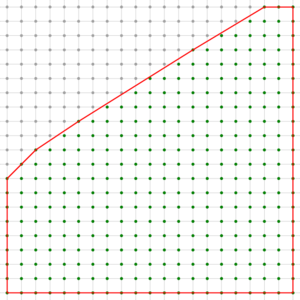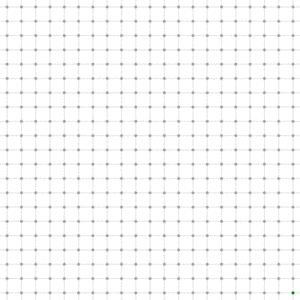DGtal  1.4.beta
arithmetic/lower-integer-convex-hull.cpp

This example shows how to create a LatticePolytope2D that represents a square. Then given integer parameters a, b, c, it cuts the polygon by the half-plane $$ax+by \le c$$. It also outputs some statistics.

Cutting the polygon by half-planes
$./examples/arithmetic/lower-integer-convex-hull -5 8 41 Number of vertices = 8 Area = 299.5 Number of interior points = 273 Number of boundary points = 55 # The animation below was created by this script (using convert and animate tools from ImageMagick package)$ let x=130; while test $x -ge -130; do echo "---$x ---"; ./examples/arithmetic/lower-integer-convex-hull -5 8 $x; let z=x+130; y=printf "%03d"$z; convert -resize 300x300 -background white -layers flatten lower-integer-convex-hull-cut.eps lower-integer-convex-hull-$y.gif; let x=x-2; done ...$ convert -delay 20 -loop 0 lower-integer-convex-hull-*.gif lower-animation.gif
\$ animate lower-animation.gifSquare of side 20 cut by the half-plane -5x+8y <= 41Square cut by the half space -5x+8y <= c, for c from -130 to 130
#include "DGtal/base/Common.h"
#include "DGtal/arithmetic/LatticePolytope2D.h"
#include "DGtal/io/boards/Board2D.h"
#include "DGtal/shapes/Shapes.h"
#include "DGtal/helpers/StdDefs.h"
using namespace DGtal;
void usage( int, char** argv )
{
std::cerr << "Usage: " << argv[ 0 ] << " <a> <b> <c>" << std::endl;
std::cerr << "\t - Cuts a square by ax+by <= c. Displays everything in files "
<< "lower-integer-convex-hull*.eps" << std::endl;
}
int main( int argc, char** argv )
{
if ( argc < 4 )
{
usage( argc, argv );
return 0;
}
using namespace Z2i;
typedef LatticePolytope2D<Space> CIP;
CIP cip;
cip.push_front( Point( -10, -10 ) );
cip.push_front( Point( -10, 10 ) );
cip.push_front( Point( 10, 10 ) );
cip.push_front( Point( 10, -10 ) );
Domain domain = cip.boundingBoxDomain();
Board2D board;
board << domain
<< CustomStyle( cip.className(),
new CustomColors( Color::Red, Color::None ) )
<< cip;
board.saveEPS( "lower-integer-convex-hull.eps" );
board.clear();
int a = atoi( argv[ 1 ] );
int b = atoi( argv[ 2 ] );
int c = atoi( argv[ 3 ] );
HalfSpace hs( Vector( a, b ), c );
cip.cut( hs );
DigitalSet aSet( domain );
board << domain
<< CustomStyle( aSet.className(),
new CustomColors( Color::Green, Color::Green ) )
<< SetMode( Point().className(), "Grid" )
<< aSet
<< CustomStyle( cip.className(),
new CustomColors( Color::Red, Color::None ) )
<< cip;
board.saveEPS( "lower-integer-convex-hull-cut.eps" );
std::cout << "Number of vertices = " << cip.size() << std::endl;
std::cout << "Area = " << (((double)cip.twiceArea())/2.0) << std::endl;
std::cout << "Number of interior points = " << cip.numberInteriorPoints() << std::endl;
std::cout << "Number of boundary points = " << cip.numberBoundaryPoints() << std::endl;
return 0;
}
void usage(int, char **argv)
static const Color None
Definition: Color.h:412
static const Color Green
Definition: Color.h:417
static const Color Red
Definition: Color.h:416
ClosedIntegerHalfPlane< Space > HalfSpace
static void makeSetFromPointPredicate(DigitalSet &aSet, const PointPredicate &aPP)
Space::Vector Vector
Definition: StdDefs.h:96
Space::Point Point
Definition: StdDefs.h:95
DGtal is the top-level namespace which contains all DGtal functions and types.
int main(int argc, char **argv)
Domain domain
HyperRectDomain< Space > Domain
Z2i::DigitalSet DigitalSet